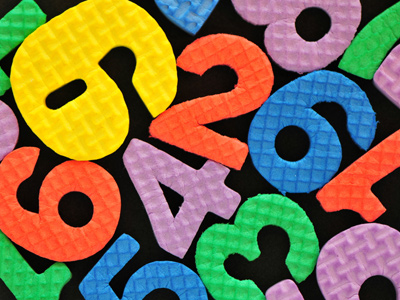You'll find a mixed bag of numbers to multiply in this times tables quiz.

# Times Tables (Mixed)

Learning the times tables is a vital tool when multiplying numbers. By the end of Year 2 children are often fluent in their times tables up to 10. When they enter into KS2 in Year 3 they will also be taught some higher times tables in their Maths and Numeracy lessons. By the end of KS2 they will be familiar with all of the times tables from one to twelve. This quiz will test their knowledge of all the different times tables.

This times tables quiz will ask you to multiply different numbers. This will help you to practise all the times tables you have been learning in your classes. Keep playing the times tables quizzes because learning the times tables by heart will make it easier to multiply different numbers together. This comes in very handy when dealing with figures in everyday life!

See how well you know your times tables by playing this quiz.

1.
8 x 11 =
80
84
88
92
To multiply a low number by 11 just use the same number twice, so 2 x 11 = 22, 4 x 11 = 44 and 8 x 11 = 88
2.
9 x 6 =
45
51
53
54
If you know your 9 times table or your 6 times table then you'll have gotten the answer straight away
3.
4 x 9 =
32
33
36
38
5 x 9 = 45 and 45 - 9 = 36
4.
7 x 7 =
49
51
54
57
5.
9 x 7 =
61
63
65
68
6.
6 x 7 =
38
42
46
48
7.
6 x 8 =
42
44
48
49
8.
5 x 8 =
40
42
45
46
To multiply a number by 5 first times it by 10 and then half it, so 8 x 10 = 80 and 80 ÷ 2 = 40
9.
8 x 8 =
54
57
64
67
A number times itself is called a square, so 8 squared is 64
10.
12 x 9 =
92
99
108
120
To multiply any number by 9 just times it by 10 and then take it away, so 12 x 10 = 120 and 120 - 12 = 108

Author:  Amanda Swift